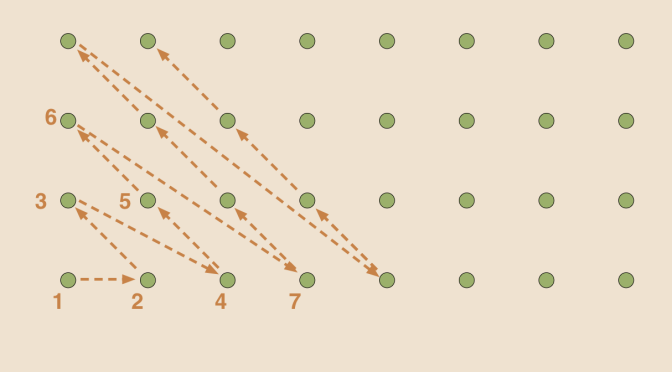# Counterexamples around cardinality (part 2)

I follow on here from the initial article on cardinality. In that article, we have seen that $$\mathbb N$$ contains an infinite countable subset whose complement is also infinite. Namely the subsets of even and odd integers.

Let’s now consider the set $$\mathbb N \times \mathbb N$$. $$\mathbb N \times \mathbb N$$ contains a countable infinite number of copies of $$\mathbb N$$, as for all $$i \in \mathbb N$$, $$\{i\} \times \mathbb N \subset \mathbb N \times \mathbb N$$. Surprisingly, it is however possible to define a bijection between $$\mathbb N \times \mathbb N$$ and $$\mathbb N$$.

### $$\mathbb N \times \mathbb N$$ is equinumerous to $$\mathbb N$$

Consider the map $\begin{array}{l|rcl} \varphi : & \mathbb N \times \mathbb N & \longrightarrow & \mathbb N\\ & (n,m) & \longmapsto & 2^{n-1} (2m-1) \end{array}$ $$\varphi$$ is one-to-one. Suppose that $$2^{n_1-1} (2m_1-1) = 2^{n_2-1} (2m_2-1)$$. If $$n_1=1$$, we must also have $$n_2=1$$ as $$2 m_1 – 1$$ is odd. That implies $$(n_1,m_1)=(n_2,m_2)$$. Let’s now suppose $$n_1 >1$$. According to Euclid’s lemma, $$2^{n_1-1}$$ divides $$2^{n_2-1}$$ as $$2^{n_1-1}$$ is even and $$2m_2 – 1$$ odd. By symmetry $$2^{n_2-1}$$ also divides $$2^{n_1-1}$$. Finally, $$2^{n_1 – 1} = 2^{n_2 – 1}$$, $$n_1 = n_2$$ and consequently $$m_1 = m_2$$.

$$\varphi$$ is also onto. $$1$$ is the image of $$(1,1)$$ under $$\varphi$$. Let’s consider $$r > 1$$ integer. According to the unique factorization theorem, $r=p_1^{r_1} p_2^{r_2} \cdots p_s^{r_s}$ where $$p_1 < \dots < p_s$$ is an increasing sequence of primes and $$r_1, \dots, r_s$$ positive integers. If $$p_1 = 2$$, we get $$r = 2^{(r_1 + 1)-1}(2 \cdot 1 - 1)$$ or $$r = 2^{(r_1 + 1)-1}(2 m - 1)$$ with $$2m - 1 = p_2^{r_2} \cdots p_s^{r_s}$$ depending on whether $$s =1$$ or $$s >1$$. And if $$p_1 > 2$$ we can write $$r = 2^{1 – 1} (2m-1)$$ with $$2m-1 = p_1^{r_1} p_2^{r_2} \cdots p_s^{r_s}$$ as $$p_1^{r_1} p_2^{r_2} \cdots p_s^{r_s}$$ is odd. We have proven that any $$r \in \mathbb N$$ has a pre-image under $$\varphi$$.

It is possible to define a bijection between $$\mathbb N$$ and $$\mathbb N \times \mathbb N$$ in a more intuitive way by “counting” the elements of $$\mathbb N \times \mathbb N$$ along diagonals as suggested in the image of the article.

To follow on, it’s time to introduce the Schröder–Bernstein theorem which states that for two sets $$E, F$$, if there exist a one-to-one map from $$E$$ to $$F$$ and a one-to-one map from $$F$$ to $$E$$, there exists a bijection from $$E$$ to $$F$$.

Based on Schröder–Bernstein theorem, we can move to next paragraph.

### $$\mathbb Q$$ is equinumerous to $$\mathbb N$$

On one hand, the map
$\begin{array}{l|rcl} \varphi_1 : & \mathbb N & \longrightarrow & \mathbb Q\\ & n & \longmapsto & n \end{array}$ is obviously one-to-one.

On the other hand the map $\begin{array}{l|rcll} \varphi_2 : & \mathbb Q & \longrightarrow & \mathbb N\\ & 0 & \longmapsto & 0 &\\ & \frac{p}{q} & \longmapsto & 2^p(2q-1) & \mathrm{gcd}(p,q)=1, p >0\\ & \frac{p}{q} & \longmapsto & 2^{-p}(2q-1)+1 & \mathrm{gcd}(p,q)=1, p <0 \end{array}$ is also one-to-one. We can therefore conclude according to Schröder–Bernstein theorem that $$\mathbb Q$$ is equinumerous to $$\mathbb N$$. This can seem surprising as $$\mathbb Q$$ is also dense in $$\mathbb R$$, which means that for any $$x < y$$ in $$\mathbb R$$, one can find $$q \in \mathbb Q$$ with $$x < q < y$$. While this is clearly not true for $$\mathbb N$$.